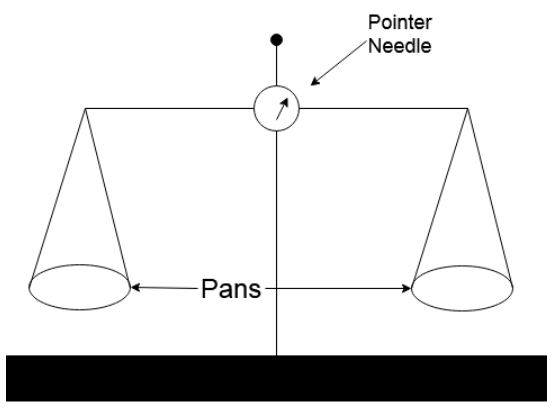QuestionAnswers

# The common balance works on the principle of equality of(A). Forces(B). Moment of forces(C). Masses(D). Masses of pansVerified
129k+ views
Hint: You can start by describing the basic structure of a common balance. Then explain how we measure the weight of the commodity by keeping the weight in both the pans of the common balance should be equal, if the weight are equal the moment of forces on both the pans are equal.